Dealing With Invalid Values

Last updated: May 22nd, 2019Dealing with Invalid values¶

After dealing with missing values, duplicated values and invalid types, our data should looks pretty good. But we'll have to do one more thing before finishing our data cleaning: checking our data domain.

Any value out of our data domain should be considered a wrong value or outlier, and be replaced or removed.Hands on!¶

In :
import numpy as np
import pandas as pd
import matplotlib.pyplot as plt
import seaborn as sns

%matplotlib inline


Let's take a look at this DataFrame:

In :
df = pd.DataFrame({
'Sex': ['M', 'F', 'F', 'D', '?', 'M'],
'Ranking': [1, 4, 2, 14, 2, 3],
'Age': [29, 30, 24, 290, 25, 18],
'Email': ['john@gmail.com', 'mary@outlook.com', 'anne.rocks',
'peter@yahoo.com', 'philip@gmail.com', 'questions@rmotr.com']
})

df

Out:
Sex Ranking Age Email
0 M 1 29 john@gmail.com
1 F 4 30 mary@outlook.com
2 F 2 24 anne.rocks
3 D 14 290 peter@yahoo.com
4 ? 2 25 philip@gmail.com
5 M 3 18 questions@rmotr.com
In :
df.dtypes

Out:
Sex        object
Ranking     int64
Age         int64
Email      object
dtype: object

No NaN values, no duplicated values, no invalid types, but...

What are the expected value domain per column?

• Sex: M for male, F for female, O for other;
• Ranking: values between 1 and 5;
• Age: values +18;
• Email: should contain a '@';

The previous DataFrame doesn't have any "missing value", but clearly has invalid data. 290 doesn't seem like a valid age, and D and ? don't correspond with any known sex category. How can you clean these not-missing, but clearly invalid values then?Finding unique values¶

The first step to clean invalid values is to notice them, then identify them and finally handle them appropriately (remove them, replace them, etc).

Sex¶

Usually, for a "categorical" type of field (like Sex, which only takes values of a discrete set ('M', 'F', 'O')), we start by analyzing the variety of values present. For that, we use the unique() method:

In :
df['Sex'].unique()

Out:
array(['M', 'F', 'D', '?'], dtype=object)

Clearly if you see values like 'D' or '?', it'll immediately raise your attention. Now, what to do with them? Let's say you picked up the phone, called the survey company and they told you that 'D' was a typo and it should actually be F. You can use the replace function to replace these values:

In :
df['Sex'].replace('D', 'F')

Out:
0    M
1    F
2    F
3    F
4    ?
5    M
Name: Sex, dtype: object

It can accept a dictionary of values to replace. For example, they also told you that there might be a few 'N's, that should actually be 'M's:

In :
df['Sex'].replace({'D': 'F', 'N': 'M'})

Out:
0    M
1    F
2    F
3    F
4    ?
5    M
Name: Sex, dtype: object

Ranking¶

Valid domain values for Ranking are numbers from 1 to 5, let's see if every value satisfies this condition:

In :
df['Ranking'].unique()

Out:
array([ 1,  4,  2, 14,  3])

We found a 14, so we need to reduce it to our maximum possible value that is 5:

In :
df['Ranking'].replace(14, 5)

Out:
0    1
1    4
2    2
3    5
4    2
5    3
Name: Ranking, dtype: int64

If we have many columns to replace, we could apply it at "DataFrame level":

In :
df.replace({
'Sex': {
'D': 'F',
'N': 'M',
'?': 'O'
},
'Ranking': {
14: 5
}
}, inplace=True)

df

Out:
Sex Ranking Age Email
0 M 1 29 john@gmail.com
1 F 4 30 mary@outlook.com
2 F 2 24 anne.rocks
3 F 5 290 peter@yahoo.com
4 O 2 25 philip@gmail.com
5 M 3 18 questions@rmotr.com
In :
fig, ax = plt.subplots(figsize=(16, 6))

sns.countplot(df['Ranking'])

Out:
<matplotlib.axes._subplots.AxesSubplot at 0x7f5344e71c50>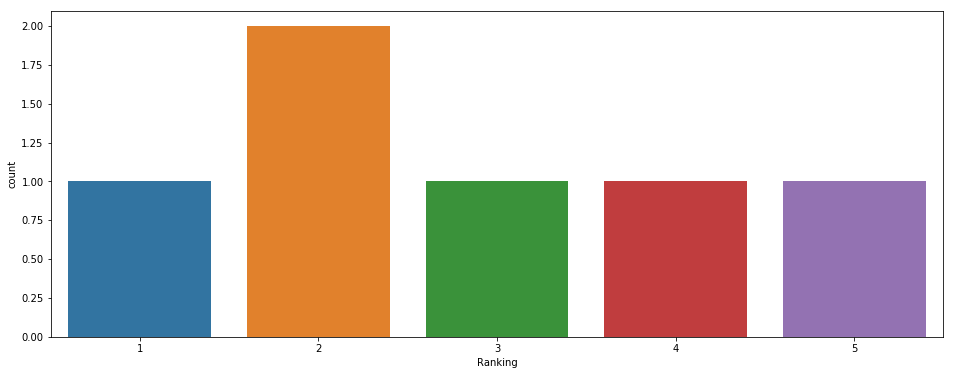Age¶

We expect numeric positive values higher than 18:

In :
df['Age'].unique()

Out:
array([ 29,  30,  24, 290,  25,  18])
In :
fig, ax = plt.subplots(figsize=(16, 6))

sns.distplot(df['Age'])

Out:
<matplotlib.axes._subplots.AxesSubplot at 0x7f5344e71780>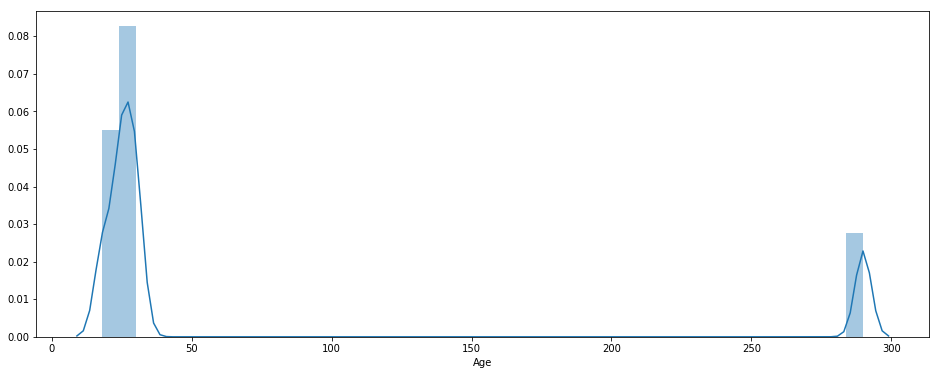In :
fig, ax = plt.subplots(figsize=(16, 6))

sns.boxplot(df['Age'])

Out:
<matplotlib.axes._subplots.AxesSubplot at 0x7f5342bcdac8>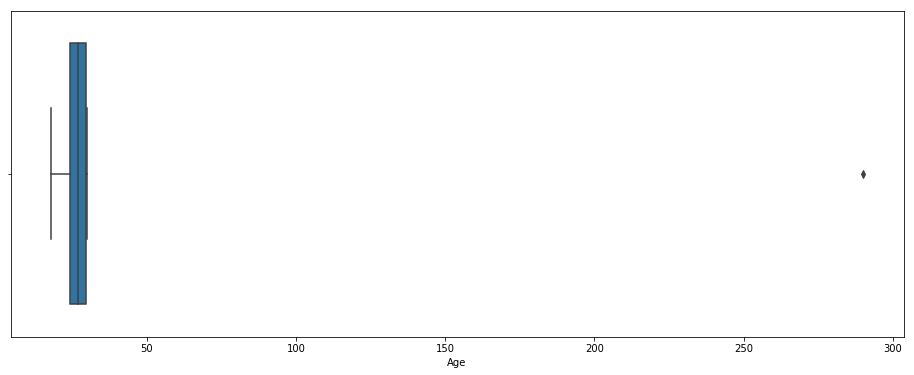Here we see a 290, that we can assume it has an extra 0 at the end, probably badly entered at data-entry phase, so it should be a 29.

But what if you'd like to remove all the extra 0s from the ages columns? (example, 150 to 15, 490 to 49).

The first step would be to just set the limit of the "not possible" age. Is it 100? 120? Let's say that anything above 100 isn't credible for our dataset. We can then combine boolean selection with the operation:

In :
df[df['Age'] > 100]

Out:
Sex Ranking Age Email
3 F 5 290 peter@yahoo.com

And we can now just divide by 10:

In :
df.loc[df['Age'] > 100, 'Age'] = (df.loc[df['Age'] > 100, 'Age'] / 10)

In :
df['Age']

Out:
0    29.0
1    30.0
2    24.0
3    29.0
4    25.0
5    18.0
Name: Age, dtype: float64
In :
fig, ax = plt.subplots(figsize=(16, 6))

sns.distplot(df['Age'])

Out:
<matplotlib.axes._subplots.AxesSubplot at 0x7f5342cce400>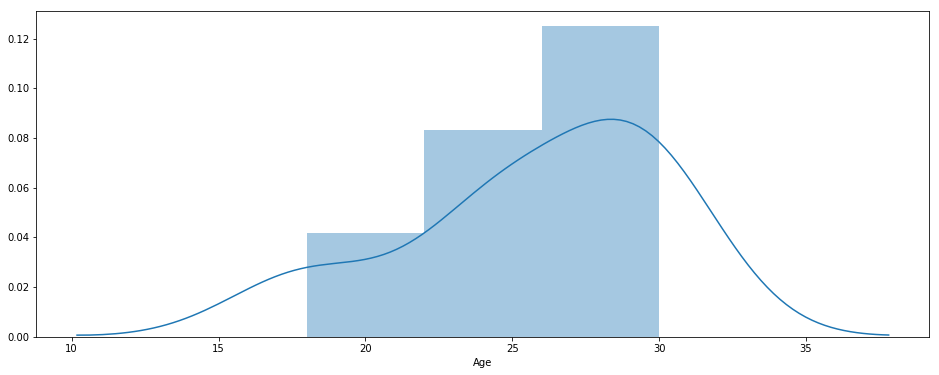In :
fig, ax = plt.subplots(figsize=(16, 6))

sns.boxplot(df['Age'])

Out:
<matplotlib.axes._subplots.AxesSubplot at 0x7f5342df1940>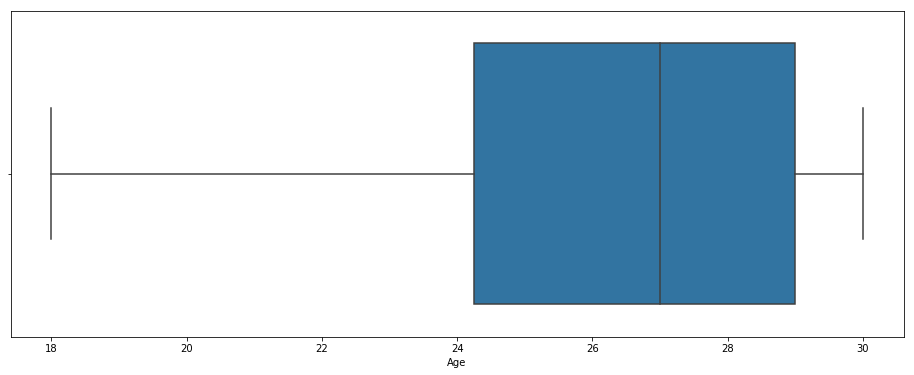Email¶

We defined that a valid email will be any string containing a @:

In :
df['Email'].unique()

Out:
array(['john@gmail.com', 'mary@outlook.com', 'anne.rocks',
'peter@yahoo.com', 'philip@gmail.com', 'questions@rmotr.com'],
dtype=object)

We have a wrong anne.rocks email, as we don't know what is it's correct domain, so we'll need to mark it as a NaN value:

In :
df['Email'].str.contains('@')

Out:
0     True
1     True
2    False
3     True
4     True
5     True
Name: Email, dtype: bool
In :
~df['Email'].str.contains('@')

Out:
0    False
1    False
2     True
3    False
4    False
5    False
Name: Email, dtype: bool
In :
df.loc[~df['Email'].str.contains('@'), 'Email'] = np.nan

In :
df['Email']

Out:
0         john@gmail.com
1       mary@outlook.com
2                    NaN
3        peter@yahoo.com
4       philip@gmail.com
5    questions@rmotr.com
Name: Email, dtype: object